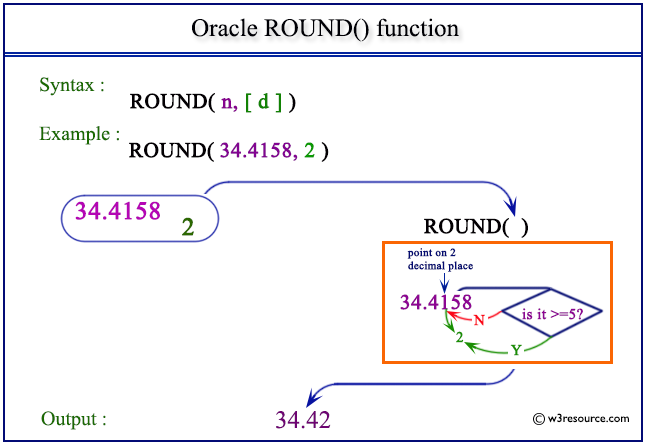# Oracle ROUND() function

## Description

This function is used to return n rounded to integer places to the right of the decimal point.

Using the following rules ROUND() function is implemented:

• If no integer is defined, then n is rounded to zero places.
• If the integer specified is negative, then n is rounded off to the left of the decimal point.
• If n is positive, then :
ROUND(n, integer) = FLOOR(n * POWER(10, integer) + 0.5) * POWER(10, -integer)

Syntax:

```ROUND(n [, D ])
```

Parameters:

Name Description
n A number which will be rounded upto D decimal places.
D A number indicating up to how many decimal places n will be rounded.

Pictorial Presentation of ROUND() functionExample:

``````SELECT ROUND(4.43) FROM dual;
```
```

Here is the result.

```ROUND(4.43)
-----------
4
```

The above statement will round the given number 4.43. No decimal places have been defined, so the default decimal value is 0.

Example: ROUND() function with negative value

``````SELECT ROUND(-4.53) FROM dual;
```
```

Here is the result.

```ROUND(-4.53)
------------
-5
```

The above statement will round the given number -4.53. No decimal places have been defined, so the default decimal value is 0.

Example: ROUND() function using decimal places

``````SELECT ROUND(-4.535,2) FROM dual;
```
```

Here is the result.

```ROUND(-4.535,2)
---------------
-4.54
```

The above statement will round the given number -4.535 up to 2 decimal places.

Example: ROUND() function using negative decimal places

``````SELECT ROUND(34.4158,-1) FROM dual;
```
```

Here is the result.

```ROUND(34.4158,-1)
-----------------
30
```

The above statement will round the given number 34.4158 from the left of decimal place up to 1 place.

Previous: REMAINDER
Next: SIGN

﻿